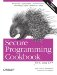# Sorting a Range

Problem

You have a range of elements that you need to sort.

Solution

There are a handful of algorithms you can use for sorting a range. You can do a conventional sort (ascending or descending order) with sort, defined in , or you can use one of the other sorting functions, such as partial_sort . Have a look at Example 7-6 to see how.

Example 7-6. Sorting

```#include
#include
#include
#include
#include
#include
#include
#include "utils.h" // For printContainer( ): see 7.10

using namespace std;

int main( ) {

cout << "Enter a series of strings: ";
istream_iterator start(cin);
istream_iterator end; // This creates a "marker"
vector v(start, end);

// The sort standard algorithm will sort elements in a range. It
// requires a random-access iterator, so it works for a vector.
sort(v.begin( ), v.end( ));
printContainer(v);

random_shuffle(v.begin( ), v.end( )); // See 7.2

string* arr = new string[v.size( )];

// Copy the elements into the array
copy(v.begin( ), v.end( ), &arr);

// Sort works on any kind of range, so long as its arguments
// behave like random-access iterators.
sort(&arr, &arr[v.size( )]);
printRange(&arr, &arr[v.size( )]);

// Create a list with the same elements
list lst(v.begin( ), v.end( ));

lst.sort( ); // The standalone version of sort won't work; you have
// to use list::sort. Note, consequently, that you
// can't sort only parts of a list.

printContainer(lst);
}```

A run of Example 7-6 might look like this:

```Enter a series of strings: a z b y c x d w
^Z
-----
a b c d w x y z
-----
w b y c a x z d
-----
a b c d w x y z
-----
a b c d w x y z```

Discussion

Sorting is a common thing, and there are two ways you can sort a sequence. You can keep elements in sorted order by using an associative container, but then you pay logarithmic time for insertions. Or, you can sort them only as needed, for which you have several options.

The sort standard algorithm does just what you'd expect: it sorts the elements in a range in ascending order using operator<. Its declaration looks like this:

```void sort(Rnd first, Rnd last);
void sort(Rnd first, Rnd last, BinPred comp);```

As with most other algorithms, you can supply your own comparison operator for sorting if operator< isn't what you want. Complexity is, in the average case, n log n. It can be quadratic in the worst case.

If you require that equivalent elements retain their relative order, use stable_sort. It has the same signature, but guarantees that equivalent elements will not have their relative order changed. Its complexity is also a little different in that it is n log n in the worst case, as long as there is memory available. If there isn't enough extra memory available, it can be at most n (log n)2.

sort doesn't work for any container, though. It requires random-access iterators, so if you are using a container that doesn't provide them, it won't work. The standard sequence containers deque, vector, and string/wstring (which are not containers, but satisfy almost all of the sequence container requirements), all provide random access iterators. list is the only one that doesn't. If you need to sort a list, you can use list::sort. For example, in Example 7-6 you will probably notice that list::sort takes no arguments:

`lst.sort( );`

This makes it distinct from std::sort, in that you can't sort only parts of a list. If you need to sort parts of a sequence, you may be better off using a sequence other than a list.

The concept of sorting is pretty straightforward, but there are a few variations on the theme that are implemented in the standard library. The following list describes each of them:

partial_sort

Takes three random-access iterators: first, middle, and last, and optionally a comparison functor. It has two postconditions: the elements in the range (first, middle) are all less than those in the range (middle, last), and the range (first, middle) is sorted according to operator< or your comparison functor. In other words, it sorts until the first n elements are sorted.

partial_sort_copy

Does the same thing as partial_sort, but places the results in an output range. It takes the first n elements from the source range and copies them into the destination range in sorted order. If the destination range (n) is shorter than the source range (m), only n items are copied into the destination range.

nth_element

Takes three random-access iterator arguments: first, nth, and last, and an optional comparison functor It puts the element referred to by nth at the index where it would be if the entire range were sorted. Consequently, all elements in the range (first, nth) are less than the element at the nth position (those in (nth, last) are not sorted, but are all greater than the ones preceding nth). You would use this if you only want one or a few elements sorted in a range, but you don't want to pay for sorting the entire range if you don't have to.

You can also partition the elements in a range according to your own criterion (functor), and that is the subject of Recipe 7.7.

Recipe 7.7Secure Programming Cookbook for C and C++: Recipes for Cryptography, Authentication, Input Validation & More
ISBN: 0596003943
EAN: 2147483647
Year: 2006
Pages: 241